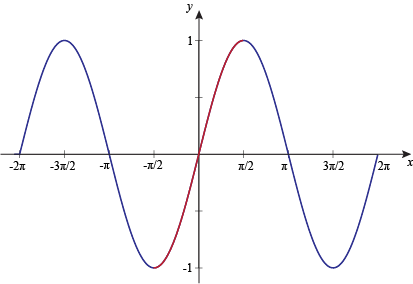5.2k views
Consider the function $f(x) = \sin(x)$ in the interval $x =\left[\frac{\pi}{4},\frac{7\pi}{4}\right]$. The number and location(s) of the local minima of this function are

1. One, at $\dfrac{\pi}{2}$
2. One, at $\dfrac{3\pi}{2}$
3. Two, at $\dfrac{\pi}{2}$ and $\dfrac{3\pi}{2}$
4. Two, at  $\dfrac{\pi}{4}$ and $\dfrac{3\pi}{2}$
in Calculus
edited | 5.2k views
+16

Local Minima :- $f(x)$ will have local minima at $x=a$ iff $f(a-h)>f(a)<f(a+h)$where $h->0$

Local Maxima :- $f(x)$ will have local maxima at $x=a$ iff  $f(a-h)<f(a)>f(a+h)$ where $h->0$

Global Minima :- $f(x)$ will have global minima at $x=a$ iff $f(x)≥f(a)$ ∀$x$∈ Domain of function.

Global Maxima :-$f(x)$ will have global maxima at $x=a$ iff $f(x)≤f(a)$ ∀$x$∈ Domain of function.

So overall we can say , when we talk about local minima/maxima we compare it with nearest points and then declare nature of function at that point but when we talk about Global minima/maxima we compare it with whole domain.

Global minima $⊆$ Local minima  and similarly Global maxima $⊆$ Local maxima+1

The answer should be B. The point at Pi/4 is not a local minima. Here's the definition from NCERT book, it should be clear that D does not satisfy the definition. I think the question was wrong and remain unchallenged, or perhaps I am wrong in which case I would love to be corrected :)0

@Divy Kala

My approach is end points can be global maximum/minimum

Also Global minima ⊆ Local minima

So if end point is can be global minima , it can also be local minima .

So the concept of end point can  be local minima is justified.

@Arjun

Sir plz tell if I 'm thinking correctly ?

0

@Apoorva Jain i guess it's a matter of convention then. the link you provide has a different definition than the ncert one i gave above. @Arjunsir please guide on this matter

edit: apparently @Arjunsir has already commented below, the answer is D according to him as well as the official key. This means NCERT definition is to be followed.

Answer is $(d)$

$f '(s) = \cos x =0$ gives root $\frac{\pi}{2}$ and $\frac{3\pi}{2}$ which lie between the given domain in question $\left[\frac{\pi}{4},\frac{7\pi}{4}\right]$

$f ''(x)= - \sin x$ at $\frac{\pi}{2}$ gives $-1<0$ which means it is local maxima and at $\frac{3\pi}{2}$ it gives $1>0$ which is local minima.

Since, at $\frac{\pi}{2}$ it is local maxima so, before it, graph is strictly increasing, so $\frac{\pi}{4}$ is also local minima.

So, there are two local minima $\frac{\pi}{4}$ and $\frac{3\pi}{2}.$

edited by
+1
+1 (y)
0

@Praveen Saini@Arjun @Bikram sir

I understand that if the endpoint is to the left of local maxima , then the endpoint is local minima and if the endpoint is to the left of local minima then the endpoint should be local maxima ... now what if the stationary point next to endpoint is an inflection point ??? Now how to find out whether endpoint is local maxima or minima ???

+4
Why pi/4 is local minima?
+1how is pi/4 even a local mimima????

+1
okay finally got it....
0
@Sushmita, Can you please explain in detail?
0
Can we say that $\frac{\pi}{4}$ is a local minima because we  have for all x in an interval $(\frac{\pi}{4},\frac{\pi}{2})$ such that $f(x)>f(\frac{\pi}{4})$  ?
+6

See the picHere it is not asked for absolute minima but it asked for local minima

local minima is the point, where the graph starts strictly increasing

Here the graph starts at $\pi /4$ and then strictly increases

So, it is one local minima

Sine function increases till $\frac{\pi}{2}$ and so for the considered interval $\frac{\pi}{4}$ would be a local minimum. From $\frac{\pi}{2}$, value of sine keeps on decresing till $\frac{3\pi}{2}$ and hence $\frac{3\pi}{2}$ would be another local minima. So, (D) is the correct answer here.
by
edited
+4

Answer is D only. 2012 onward official keys are available.

http://gatecse.in/w/images/b/b5/Key_CS_2012.pdf

+13

This is trick question. Local word matters !

+1
Why are we considering pi/4 here. They have asked for local minima and not absoulte minina, so shouldnt we consider the open interval only?
+2
what has "local" to do with interval being closed or open? [ ] means it is closed interval and ( ) means it is open interval.
0
Sir, accroding to me there should be only one minima at 3pi/2. I am under the impression that "Local" means we should consider the open interval (pi/4,7pi/4). Please correct me if I am wrong.

Definition : http://tutorial.math.lamar.edu/Classes/CalcI/MinMaxValues.aspx
0
@Arjun SIR

f(x) = sin x

f'(x) = cos x
solve for  cos x = 0  x= n (pi) / 2

f''(x) = - sin x
at  x= n (pi) / 2   f''(x) <0  implies local maxima

How did u find local minima ?
0
@arjun sir

please explain why we have consider $\prod /4$ here ??
0
Simply visualize the graph of sinx between those interval.
+2
why is pi/4 considered?
+1 vote
Simplest way possible!by
edited
+1
plz rotate the image
0
The signs are wrong. In fact, they should be opposite to what is given by you.
+1 vote

NCERT 12th class maths book pg no:228 (chapter 6):I don't think there is any doubt regarding local minima at point $c=\frac{\pi}{4}$.